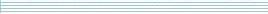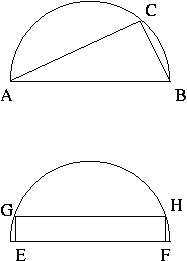| home      | people     | research     | publications    | seminars    | events     | contactCommunication Networks   | Systems Biology   | Hybrid Systems    | Machine Learning   | Dynamics & Interaction### Schools Mathematics Grand Challenge

Week seven's Puzzles

Problem 13:

The thirteenth problem was:

Take a 2 digit number `ab` (so a number between 10 and 99). Form a 10 digit number from it by placing five copies of the number next to each other. For example, if the original number is 13, we form the 10 digit number

1313131313

or if the original number is 37 we form

3737373737.

There is exactly one 2 digit number such that when you add up the digits of the ten digit number obtained in this way, the sum is equal to the original number. What is it?

The solution was:

If the digits of the original number ab, so a is in the 10s place and b in the ones place, then the original number is 10a + b. If we take 5 copies of the digits and add them up, we get 5(a + b). These must be the same, so 10a + b = 5a + 5b, or 5a = 4b.

Now, remember a and b must be whole numbers between 0 and 9. One option from here is to check what whole numbers work for a and b. Another is to see that because 5 and 4 have no common divisors a must be divisible by 4 (so a = 0, 4 or 8) and b must be divisible by 5 (so b = 0 or 5). The only combination that works out is a = 4 and b = 5, so the number is 45.

Problem 14:

The fourteenth problem was:

A googol is 1 followed by 100 zeros, or 10^100.

If you check, you'll find that N=5 is the smallest number so that N! (N factorial) is divisible by 10. What is the smallest N so that N! is divisible by a googol?

The solution was:

We're looking for a factorial that is divisible by 10 one hundred times. How many powers of 10 divide any number is determined by how many times 2 divides the number and how many times 5 divides the number, because 2 and 5 are the prime factors of 10. For example, 600 has two zeros at the end and 600 = 2*2*2*3*5*5, so we can make two powers of 10 by taking 2 of the 2s and 2 of the 5s. The extra 2 that is left is no good to us, because we can't pair it up with a 5.

So, lets try to figure out how many times 2 divides into n! by making a table.

```	Factorial       Extra powers of 2                   Total
---------------------------------------------------------
1! = 1		zero powers of 2			0
2! = 1! x 2 	one extra power of 2			1
3! = 2! x 3 	no extra power of 2			1
4! = 3! x 4 	4 = 2*2, so 2 extra powers of 2		3
5! = 4! x 5	no extra powers of 2			3
6! = 5! x 6	6 = 3*2, so 1 extra power of 2		4
7! = 6! x 7	no extra powers of 2			4
8! = 7! x 8	8 = 2*2*2, so 3 extra powers of 2	7
```

The pattern is that that we get 1 power if the number is even, 2 if it is divisible by 4, 3 if it is divisible by 8 and so on. However, 4 is even, so we're really only getting 1 extra. And 8 is divisible by 4, so we're really only getting another one extra.

So, we have to count the even numbers less than n - this is easy there are [n/2] of them, but we must round down. Then we need to count the numbers divisible by 4, this is [n/4]. So, the number of powers of two that divide n! is:

[n/2] + [n/4] + [n/8] + [n/16] + [n/32] + ...

where we round down each division. In exactly the same way we can figure out the powers of 5 that divide into n! as

[n/5] + [n/25] + [n/125] + [n/625] + [n/3125] + ...

To get a googol to divide into n!, we need these to be at least 100. The numbers of powers of two will be much bigger, so we we can just work out the number of powers of 5. Guessing n=500 we see it is a bit too big:

[500/5] + [500/25] + [500/125] + [500/625] + ... =
100 + 20 + 4 + 0 + ... =
124

So we should move back a bit. Let's try 400:

[400/5] + [400/25] + [400/125] + [400/625] + ... =
80 + 16 + 3 + 0 + ... =
99

So it is just a tiny bit too small. We need one more power of 5, which we know we'll get at the next number divisible by 5, which is 405. So this is our answer.

If you are curious 405! is way bigger than normal calculators can deal with. You can download the factorial of 404 and 405 here if you want to count the zeros for yourself!

Problem 15:

The fifteenth problem was:

You inherit a 4 hectare semicircular plot of land in the centre of Dublin from an eccentric relative. A property developer offers to buy some land from you, however they are only interested in either triangular or rectangular plots of land. They will pay you 250,000 euro per hectare of land.Look at the two plots of land above. Your first option is to sell the triangle of land ABC. AB is the diameter of the semicircle and you can put C anywhere on the perimeter of the semicircle.

The second option is to sell a rectangle of land EFHG. You can put E and F anywhere on the base of the semicircle and G and H anywhere on the perimeter as long as EFHG is a rectangle.

What is the ratio of the value of the biggest triangular plot compared to the value of the biggest rectangular plot? Give your answer to 3 decimal places.

Hint 1: Remember your formulas for area.

Hint 2: On page 9 of the tables book you can find the formula: 2 cos(A) sin(A) = sin(2A). This means that the sine of twice an angle is equal to 2 times the product of the sine and cosine of the original angle.

The solution was:

There are at least two ways to do this puzzle. First, lets do the long way.

We want work out the area of the biggest triangle and the biggest rectangle. Let's work in terms of the radius of the circle, and call that r.

The area of a triangle is 1/2 x (base) x (height). Our triangle has a fixed base = 2r. The only thing that we can change is where point C is, which changes the height. To make the area as big as possible, we make the height as big as possible, which happens when C is directly above the centre, so the height will be r. So, the area of the biggest triangle is 1/2 x (2r) x (r) = r^2. Here's a picture.For the rectangle, join up the centre of the circle to corner G. We now can see a right angle triangle, with an angle A between the base of the semicircle and the line to G. We can see that the height of our rectangle is (r Sin A) and the length is (2r Cos A), so the area of the rectangle is (r^2 2 Sin A Cos A). By the hint this is (r^2 Sin 2A). Remember that biggest Sin X can be is 1, when X = 90. So we get the biggest rectangle when 2A = 90, or A = 45. The area of this rectangle will be (r^2 x Sin 90) = r^2.

So, we see that the areas are the same and the ratio is 1.

Now, the short way. Imagine reflecting the triangle through the centre of the circle and the rectangle through the base of the semicircle. We end up with a picture like this.In both cases we end up with a rectangle of twice the area of our original figure inscribed in a circle. The problem of making the biggest area in both cases is the same, so the ratio must be 1.